# Fraction calculator

The calculator performs basic and advanced operations with fractions, expressions with fractions combined with integers, decimals, and mixed numbers. It also shows detailed step-by-step information about the fraction calculation procedure. Solve problems with two, three, or more fractions and numbers in one expression.

## Result:

### 4 : (1/5) = 20/1 = 20

Spelled result in words is twenty.

### How do you solve fractions step by step?

1. Divide: 4 : 1/5 = 4/1 · 5/1 = 4 · 5/1 · 1 = 20/1 = 20
Dividing two fractions is the same as multiplying the first fraction by the reciprocal value of the second fraction. The first sub-step is to find the reciprocal (reverse the numerator and denominator, reciprocal of 1/5 is 5/1) of the second fraction. Next, multiply the two numerators. Then, multiply the two denominators.
In words - four divided by one fifth = twenty.

#### Rules for expressions with fractions:

Fractions - use the slash “/” between the numerator and denominator, i.e., for five-hundredths, enter 5/100. If you are using mixed numbers, be sure to leave a single space between the whole and fraction part.
The slash separates the numerator (number above a fraction line) and denominator (number below).

Mixed numerals (mixed fractions or mixed numbers) write as non-zero integer separated by one space and fraction i.e., 1 2/3 (having the same sign). An example of a negative mixed fraction: -5 1/2.
Because slash is both signs for fraction line and division, we recommended use colon (:) as the operator of division fractions i.e., 1/2 : 3.

Decimals (decimal numbers) enter with a decimal point . and they are automatically converted to fractions - i.e. 1.45.

The colon : and slash / is the symbol of division. Can be used to divide mixed numbers 1 2/3 : 4 3/8 or can be used for write complex fractions i.e. 1/2 : 1/3.
An asterisk * or × is the symbol for multiplication.
Plus + is addition, minus sign - is subtraction and ()[] is mathematical parentheses.
The exponentiation/power symbol is ^ - for example: (7/8-4/5)^2 = (7/8-4/5)2

#### Examples:

subtracting fractions: 2/3 - 1/2
multiplying fractions: 7/8 * 3/9
dividing Fractions: 1/2 : 3/4
exponentiation of fraction: 3/5^3
fractional exponents: 16 ^ 1/2
adding fractions and mixed numbers: 8/5 + 6 2/7
dividing integer and fraction: 5 ÷ 1/2
complex fractions: 5/8 : 2 2/3
decimal to fraction: 0.625
Fraction to Decimal: 1/4
Fraction to Percent: 1/8 %
comparing fractions: 1/4 2/3
multiplying a fraction by a whole number: 6 * 3/4
square root of a fraction: sqrt(1/16)
reducing or simplifying the fraction (simplification) - dividing the numerator and denominator of a fraction by the same non-zero number - equivalent fraction: 4/22
expression with brackets: 1/3 * (1/2 - 3 3/8)
compound fraction: 3/4 of 5/7
fractions multiple: 2/3 of 3/5
divide to find the quotient: 3/5 ÷ 2/3

The calculator follows well-known rules for order of operations. The most common mnemonics for remembering this order of operations are:
PEMDAS - Parentheses, Exponents, Multiplication, Division, Addition, Subtraction.
BEDMAS - Brackets, Exponents, Division, Multiplication, Addition, Subtraction
BODMAS - Brackets, Of or Order, Division, Multiplication, Addition, Subtraction.
GEMDAS - Grouping Symbols - brackets (){}, Exponents, Multiplication, Division, Addition, Subtraction.
Be careful, always do multiplication and division before addition and subtraction. Some operators (+ and -) and (* and /) has the same priority and then must evaluate from left to right.

## Fractions in word problems:

• Product of two fractionsProduct of two fractions is 9 3/5 . If one of the fraction is 9 3/7. Find the other fraction.
• Soup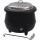On Monday we cook 25 pots and 10 boilers of soup. On Tuesday 15 pots and 13 boilers. On Wednesday 20 pots and on Thursday 30 boilers. On Monday and Tuesday was cooked the same amount of soup. How many times more soup cooked on Thursday than on Wednesday?
• ExpressionsLet k represent an unknown number, express the following expressions: 1. The sum of the number n and two 2. The quotient of the numbers n and nine 3. Twice the number n 4. The difference between nine and the number n 5. Nine less than the number n
• The thirdThe one-third rod is blue, one-half of the rod is red, the rest of the rod is white and measures 8 cm. How long is the whole rod?
• Sewing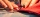Beth's mother can sew 235 pairs of short pants in 6 days while Lourdes can sew 187 pairs in 8 days. How many more pairs of short pants can Beth's mother sew?
• University bubbleYou'll notice that the college up slowly every other high school. In Slovakia/Czech republic a lot of people studying political science, mass media communication, social work, many sorts of management MBA. Calculate how many times more earns clever 25-yea
• Cooks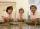Four cooks cleaned 5 kg of potatoes for 10 minutes. How many cook would have to work clean 9 kg of potatoes for 12 minutes?
• Metallurgical enterprise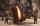Metallurgical company daily produces 318 tons of cast iron. How many tons of coke it burns to melt cast iron when 10 tons of cast consumes 6 tons of coke?
• Cathy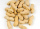Cathy has 5 cups of peanuts to share with her family. If each family member gets 1/2 cup of peanuts, how many family members can have some?
• AlmondsRudi has 4 cups of almonds. His trail mix recipe calls for 2/3 cup of almonds. How many batches of trail mix can he make?
• What is 11What is the quotient of Three-fifths and 1 Over 10?
• Jacob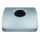Jacob is baking chocolate cookies. The recipe uses 2/8 of a cup of sugar to bake a batch of cookies. If Jacob wants to make one-half of a batch. How many cups of sugar does he need?
• Simple equation 2Find X in this simple equation: X/9 = 96/108